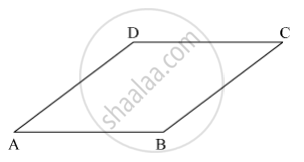SSC (English Medium) Class 8Maharashtra State Board
Share

# Measures of Opposite Angles of a Parallelogram Are (3x − 2)° and (50 − X)° . Find the Measure of Its Each Angle. - SSC (English Medium) Class 8 - Mathematics

ConceptKinds of Quadrilaterals Concept of Kite

#### Question

Measures of opposite angles of a parallelogram are (3x − 2)° and (50 − x)° . Find the measure of its each angle.

#### Solution

Let ABCD be the parallelogram.Suppose ∠A = (3x − 2)º and ∠C = (50 − x)º.

We know that the opposite angles of a parallelogram are congruent.

∴ m∠A = m∠C

⇒ 3x − 2 = 50 − x

⇒ 3x + x = 50 + 2

⇒ 4x = 52

⇒ x = 13

∴ m∠A = (3x − 2)º = (3 × 13 − 2)º= (39 − 2)​º= 37º

So, m∠C = m∠A = 37º

Also, the adjacent angles of a parallelogram are supplementary.

∴ m∠A + m∠D = 180º

⇒ 37º + m∠D = 180º

⇒ m∠D = 180º − 37º = 143º

Now,

m∠B = m∠D = 143º               (Opposite angles of a parallelogram are congruent)

Thus, the measure of the angles of the parallelogram are 37º, 143º, 37º and 143º.

Is there an error in this question or solution?

#### APPEARS IN

Balbharati Solution for Balbharati Class 8 Mathematics (2019 to Current)
Chapter 8: Quadrilateral : Constructions and Types
Practice Set 8.3 | Q: 1 | Page no. 49
Solution Measures of Opposite Angles of a Parallelogram Are (3x − 2)° and (50 − X)° . Find the Measure of Its Each Angle. Concept: Kinds of Quadrilaterals - Concept of Kite.
S